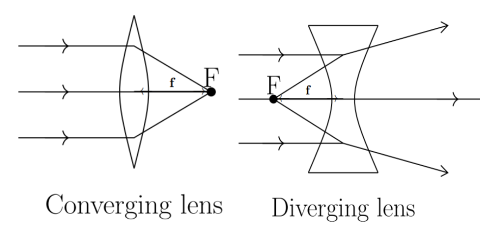Courses
Courses for Kids
Free study material
Free LIVE classes
More# Converging and diverging of light rays is the property for which of the given pairs A. Concave lens and Plane mirror respectivelyB. Convex lens and Plane mirror respectivelyC. Concave lens and convex lens respectivelyD. Convex lens and concave lens respectivelyVerified
216.3k+ views
Hint: A concave lens is a lens that possesses at least one surface that curves inwards. It spreads out light rays that have been refracted through it. Whereas a convex lens is a lens that converges the incident rays towards the principal axis after refraction through a point called focus, since it converges all the ray at one point.